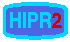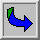# Pixel DivisionCommon Names: Pixel Division, Ratioing

## Brief Description

The image division operator normally takes two images as input and produces a third whose pixel values are just the pixel values of the first image divided by the corresponding pixel values of the second image. Many implementations can also be used with just a single input image, in which case every pixel value in that image is divided by a specified constant.

## How It Works

The division of two images is performed in the obvious way in a single pass using the formula: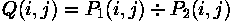Division by a constant is performed using: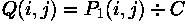If the pixel values are actually vectors rather than scalar values (e.g. for color images) than the individual components (e.g. red, blue and green components) are simply divided separately to produce the output value.

The division operator may only implement integer division, or it may also be able to handle floating point division. If only integer division is performed, then results are typically rounded down to the next lowest integer for output. The ability to use images with pixel value types other than simply 8-bit integers comes in very handy when doing division.

## Guidelines for Use

One of the most important uses of division is inchange detection, in a similar way to the use of subtraction for the same thing. Instead of giving the absolute change for each pixel from one frame to the next, however, division gives the fractional change or ratio between corresponding pixel values (hence the common alternative name ofratioing). The imagesandare of the same scene except two objects have been slightly moved between the exposures. Dividing the former by the latter using a floating point pixel type and then contrast stretching the resulting image yields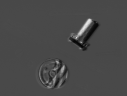After the division, pixels which didn't change between the exposures have a value of 1, whereas if the pixel value increased after the first exposure the result of the division is clustered between 0 and 1, otherwise it is between 1 and 255 (provided the pixel value in the second image is not smaller than 1). That is the reason why we can only see the new position of the moved part in the contrast-stretched image. The old position can be visualized by histogram equalizing the division output, as shown in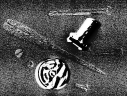Here, high values correspond to the new position, low values correspond to the old position, assuming that the intensity of the moved object is lower than the background intensity. Intermediate graylevels in the equalized image correspond to areas of no change. Due to noise, the image also shows the position of objects which were not moved.

For comparison, the absolute difference between the two images, as shown inproduces approximately the same pixel values at the old and the new position of a moved part.Another application for pixel division is to separate the actual reflectance of an object from the unwanted influence of illumination. This image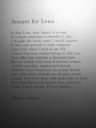shows a poorly illuminated piece of text. There is a strong illumination gradient across the image which makes conventional foreground/background segmentation using standard thresholding impossible. The imageshows the result of straightforward intensity thresholding at a pixel value of 128. There is no global threshold value that works over the whole of the image.

Suppose that we cannot change the lighting conditions, but that we can take several images with different items in the viewfield. This situation arises quite a lot in microscopy, for instance. We choose to take a picture of a blank sheet of white paper which should allow us to capture the incident illumination variation. This lightfield image is shown inNow, assuming that we are dealing with a flat scene here, with points on the surface of the scene described by coordinates x and y, then the reflected light intensity B(x,y) depends upon the reflectance R(x,y) of the scene at that point and also on the incident illumination I(x,y) such that:Using subscripts to distinguish the blank (lightfield) image and the original image, we can write:But since I(x,y) is the same for both images, and assuming the reflectance of the blank paper to be uniform over its surface, then: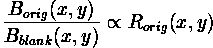Therefore the division should allow us to segment the letters out nicely. In image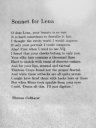we see the result of dividing the original image by the lightfield image. Note that floating point format images were used in the division, which were then normalized to 8-bit integers for display. Virtually all the illumination gradient has been removed. The image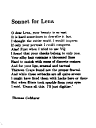shows the result of thresholding this image at a pixel value of 160. While not fantastic, with a little work using morphological operations, the text could become quite legible. Compare the result with that obtained using subtraction.

As with other image arithmetic operations, it is important to be aware of whether the implementation being used does integer or floating point arithmetic. Dividing two similar images, as done in the above examples, results mostly in very small pixel values, seldom greater than 4 or 5. To display the result, the image has to be normalized to 8-bit integers. However, if the division is performed in an integer format the result is quantized before the normalization, hence a lot of information is lost. Image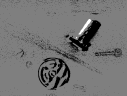shows the result of the above change detection if the division is performed in integer format. The maximum result of the division was less than 3, therefore the integer image contains only three different values, i.e. 0, 1 and 2 before the normalization. One solution is to multiply the first image (the numerator image) by a scaling factor before performing the division. Of course this is not generally possible with 8-bit integer images since significant scaling will simply saturate all the pixels in the image. The best method is, as was done in the above examples, to switch to a non-byte image type, and preferably to a floating point format. The effect is that the image is not quantized until the normalization and therefore the result does contain more graylevels. If floating point cannot be used, then use, say, 32-bit integers, and scale up the numerator image before dividing.

## Interactive Experimentation

You can interactively experiment with this operator by clicking here.

## Exercises

1. Take two images of an (analogue) watch at different times, without moving it in between. Use division to detect the change in the image and compare the result with the one achieved with pixel subtraction. How easily can one detect small motions (i.e. minutes hand vs seconds hand)?
2. Describe a possible application where determining the percentage change between two images of similar scenes using ratioing is a better idea than determining the difference between the images using subtraction.

## References

E. Davies Machine Vision: Theory, Algorithms and Practicalities, Academic Press, 1990, Chap 2.

A. Marion An Introduction to Image Processing, Chapman and Hall, 1991, p 244.

## Local Information